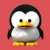## JavaScript 全文搜索之相关度评分已翻译 100%

oschina 投递于 2015/03/23 08:03 (共 12 段, 翻译完成于 03-30)

## Okapi BM25

Okapi BM25 是到目前为止被认为最先进的排名算法之一（所以被称为 ElasticSearch ）。Okapi BM25 在 TF-IDF 的基础上增加了两个可调参数，k1 和 b，, 分别代表 “词语频率饱和度（term frequency saturation）” 和 “字段长度规约”。这是什么鬼？

## 算法

Okapi BM25 维基百科中你可以了解Okapi算法的公式。既然都知道了式子中的每一项是什么，这肯定是很容易地就理解了。所以我们就不提公式，直接进入代码：

```BM25.Tokenize = function(text) {
text = text
.toLowerCase()
.replace(/\W/g, ' ')
.replace(/\s+/g, ' ')
.trim()
.split(' ')
.map(function(a) { return stemmer(a); });

// Filter out stopStems
var out = [];
for (var i = 0, len = text.length; i < len; i++) {
if (stopStems.indexOf(text[i]) === -1) {
out.push(text[i]);
}
}

return out;
};```

```BM25.prototype.addDocument = function(doc) {
if (typeof doc.id === 'undefined') { throw new Error(1000, 'ID is a required property of documents.'); };
if (typeof doc.body === 'undefined') { throw new Error(1001, 'Body is a required property of documents.'); };

// Raw tokenized list of words
var tokens = BM25.Tokenize(doc.body);

// Will hold unique terms and their counts and frequencies
var _terms = {};

// docObj will eventually be added to the documents database
var docObj = {id: doc.id, tokens: tokens, body: doc.body};

// Count number of terms
docObj.termCount = tokens.length;

// Increment totalDocuments
this.totalDocuments++;

this.totalDocumentTermLength += docObj.termCount;
this.averageDocumentLength = this.totalDocumentTermLength / this.totalDocuments;

// Calculate term frequency
// First get terms count
for (var i = 0, len = tokens.length; i < len; i++) {
var term = tokens[i];
if (!_terms[term]) {
_terms[term] = {
count: 0,
freq: 0
};
};
_terms[term].count++;
}

// Then re-loop to calculate term frequency.
// We'll also update inverse document frequencies here.
var keys = Object.keys(_terms);
for (var i = 0, len = keys.length; i < len; i++) {
var term = keys[i];
// Term Frequency for this document.
_terms[term].freq = _terms[term].count / docObj.termCount;

// Inverse Document Frequency initialization
if (!this.terms[term]) {
this.terms[term] = {
n: 0, // Number of docs this term appears in, uniquely
idf: 0
};
}

this.terms[term].n++;
};

// Calculate inverse document frequencies
// This is SLOWish so if you want to index a big batch of documents,
// comment this out and run it once at the end of your addDocuments run
// If you're only indexing a document or two at a time you can leave this in.
// this.updateIdf();

// Add docObj to docs db
docObj.terms = _terms;
this.documents[docObj.id] = docObj;
};```

this.documentsis 是一个保存着所有文档的数据库，它保存着文档的全部原始文字，文档的长度信息和一个列表，列表里面保存着文档中的所有词语和词语的数量与出现频率。使用这个数据结构，我们可以很容易的和快速的（是的，非常快速，只需要时间复杂度为O(1)的哈表查询时间）回答如下问题：在文档 #3 中，'walk' 这个词语出现了多少次？

```BM25.prototype.updateIdf = function() {
var keys = Object.keys(this.terms);
for (var i = 0, len = keys.length; i < len; i++) {
var term = keys[i];
var num = (this.totalDocuments - this.terms[term].n + 0.5);
var denom = (this.terms[term].n + 0.5);
this.terms[term].idf = Math.max(Math.log10(num / denom), 0.01);
}
};```

```BM25.prototype.search = function(query) {

var queryTerms = BM25.Tokenize(query);
var results = [];

// Look at each document in turn. There are better ways to do this with inverted indices.
var keys = Object.keys(this.documents);
for (var j = 0, nDocs = keys.length; j < nDocs; j++) {
var id = keys[j];
// The relevance score for a document is the sum of a tf-idf-like
// calculation for each query term.
this.documents[id]._score = 0;

// Calculate the score for each query term
for (var i = 0, len = queryTerms.length; i < len; i++) {
var queryTerm = queryTerms[i];

// We've never seen this term before so IDF will be 0.
// Means we can skip the whole term, it adds nothing to the score
// and isn't in any document.
if (typeof this.terms[queryTerm] === 'undefined') {
continue;
}

// This term isn't in the document, so the TF portion is 0 and this
// term contributes nothing to the search score.
if (typeof this.documents[id].terms[queryTerm] === 'undefined') {
continue;
}

// The term is in the document, let's go.
// The whole term is :
// IDF * (TF * (k1 + 1)) / (TF + k1 * (1 - b + b * docLength / avgDocLength))

// IDF is pre-calculated for the whole docset.
var idf = this.terms[queryTerm].idf;
// Numerator of the TF portion.
var num = this.documents[id].terms[queryTerm].count * (this.k1 + 1);
// Denomerator of the TF portion.
var denom = this.documents[id].terms[queryTerm].count
+ (this.k1 * (1 - this.b + (this.b * this.documents[id].termCount / this.averageDocumentLength)));

// Add this query term to the score
this.documents[id]._score += idf * num / denom;
}

if (!isNaN(this.documents[id]._score) && this.documents[id]._score > 0) {
results.push(this.documents[id]);
}
}

results.sort(function(a, b) { return b._score - a._score; });
return results.slice(0, 10);
};```

### 评论(12)http://www.oschina.net/news/61153/xunsearch-1-4-9

【性能劲爆】单库最多支持 40 亿条数据，在 5 亿网页大约 1.5TB 的数据中检索时间不超过 1 秒（非缓存）。

【简单易用】前端是使用脚本语言 PHP 编写的开发工具。API 简单清晰，开发难度极低，提供全中文的示例代码、文档、辅助脚本工具等。

【功能丰富】除支持基础的自定义分词、字段检索、布尔搜索外，还直接支持用户急需的相关搜索、拼音搜索、搜索建议等专业功能。

XunSearch作者同时是中文分词SCWS的作者hightman。